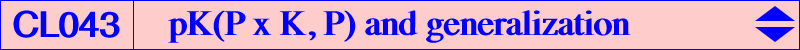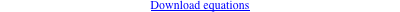Any pK with pivot P=u:v:w, isopivot K (Lemoine point) and pole W = P x K (barycentric product) meets the circumcircle at A, B, C (where the tangents are the symmedians) and three other points Q1, Q2, Q3 (not necessarily all real) where the tangents are concurrent at a point X. The first barycentric coordinate of X is : a^2 [2 u v w (–13 b^2 c^2 u + a^2 c^2 v + a^2 b^2 w) + a^4 v^2 w^2 – 3 u^2 (c^4 v^2 + b^4 w^2)] This type of pK is the isogonal transform of a pK with pivot G and pole the isogonal conjugate of P. The pK always contains P, K, P/K (cevian quotient) and the crossconjugate of K and P (the isoconjugate of P/K). Locus property : Let S be the P–Ceva conjugate of K i.e. the perspector of the cevian triangle of P and the tangential triangle. The locus of M such that the anticevian triangle of M and the circumcevian triangle of S are perspective (at Q) is the pK above. The locus of Q is another pK with same pole and pivot the barycentric product of the S–Ceva conjugate of K and tgP (isotomic of isogonal of P). A simple example : with P = X(2) hence S = X(3), the two pKs above are both isogonal cubics, namely K002 and K004 respectively.Special pK(P x K, P)Circular cubic The only circular cubic is obtained with P = X(671) and the pole is W = X(111). This is K273. The two isotropic tangents meet at the singular focus F which lies on the tangent at X(111). F is X(11258), the reflection of O in X(111). *** Equilateral cubic The only pK60 is K375. It is obtained when P is the isotomic conjugate of the intersection of the lines X(3)X(523) and X(315)X(524). The three tangents at Q1, Q2, Q3 make 60° angles with one another. *** pK+ The cubic has three concurring asymptotes (at X) if and only if P lies on a circumcubic passing through K (the cubic decomposes into the union of the symmedians), X(141), X(193), X(2998), the infinite points of pK(X39, X6), the reflections of the cevians of X(76) in the corresponding cevians of X(6). This gives the cubics pK(X39, X141) and pK(X3053, X193). The locus of X is a cubic passing through X(2), X(6), X(3167), the infinite points of pK(X3, X6), the cevians of X(6). *** Cubics passing through the pole of isoconjugation Every cubic K(P) = pK(P x K, P) with P on the Kiepert hyperbola contains its pole W = P x K and also G and H. The third point on the Euler line is the P-Ceva conjugate of X6. This is the case of the Thomson cubic K002 and several other cubics shown in the following table.pivot centers on the cubic : X2, X4, X6 and Xi or simply i cubic X2 1, 3, 9, 57, 223, 282, 1073, 1249, 3341, 3342, 3343, 3344, 3349, 3350, 3351, 3352, 3356, 14481, 39162, 39163, 39164, 39165, 40989, 40990, 40991, 40992 K002 X4 25, 193, 371, 372, 2362, 7133, 14248, 16232, 20034, 41515, 41516, 42013 K233 X10 X10, X42, X71, X199, X1654 X13 13, 15, 62, 1251, 2306, 3129, 3180, 3457, 8015, 11080, 11082, 19551, 33655, 36296, 36298, 36300, 36302, 36304 K1145a X14 14, 16, 61, 3130, 3181, 3458, 7052, 7126, 8014, 11085, 11087, 33653, 33654, 36297, 36299, 36301, 36303, 36305 K1145b X76 22, 69, 76, 1670, 1671, 18018, 19613, 41361 K141 X83 83, 251, 1176, 1342, 1343, 8743, 40357, 40358, 40404 K644 X94 X94, X265, X1989, X2070 X98 98, 237, 248, 385, 1687, 1688, 1976, 34238, 34536, 41204 K380 X226 X73, X226, X1400 X262 262, 263, 1689, 1690, 3148, 7774, 14251, 41371, 43718 K791 X275 X54, X275, X1993 X321 X37, X72, X321, X2895, X2915 X598 598, 1383, 1992, 1995, 14246, 41370, 43697 K283 X671 23, 111, 524, 671, 895, 5523, 10415, 10630, 13492, 14262, 39229, 39230, 41498, 41511 K273 X801 X20, X394, X801 X1446 X1427, X1439, X1446 X1916 39, 256, 291, 511, 694, 1432, 1916, 3978, 4876, 6660, 7779, 9230, 9469, 14946, 14970, 21355, 24479, 36214, 36897, 40873 K354 X2052 24, 393, 847, 2052, 6515 K621 X2394 X2394, X2433 X2592 X2574, X2592 X2593 X2575, X2593 X2986 X30, X323, X2986 X3407 32, 182, 983, 985, 2344, 3114, 3407, 7132, 7766, 11328, 18898, 32544, 40820, 43722 K1013 X14534 21, 58, 81, 572, 961, 1169, 1220, 1798, 2298, 14534, 19607, 41364 K379 X16080 74, 186, 1990, 3580, 5627, 8749, 11070, 16080, 40384, 40385, 40386, 40387, 40388, 40389, 40390, 40391, 40392 K1172 X34258 8, 386, 573, 941, 959, 2345, 5739, 11337, 34258, 34259, 34260, 34262, 34263, 34264, 34265, 34266, 34267, 34268, 34269, 34270, 34271, 34272, 34273, 34274, 34275, 34276, 34277 K1135 X40395 28, 284, 580, 943, 1172, 1175, 2982, 40395, 40570, 40571, 40572, 40573, 40574, 40575 K1174Remarks : Every such cubic K(P) meets the line at infinity and the circumcircle again at the same points as two isogonal pKs with pivots tP and atP respectively. The tangents at the three points on the circumcircle are concurrent and the three lines passing through these points envelope the parabola (P) with focus X(111) and directrix the line GK. (P) passes through X{1499, 1640, 3288, 5915, 9209}. The dual conic of (P) is a rectangular hyperbola (H), homothetic to the Kiepert hyperbola, passing through X{2, 20, 69, 76, 99, 3413, 3414, 3663, 6337, 9772, 11057, 20880, 22339, 22340, 32476}. Its center is the midpoint of X(69), X(99), also the reflection of X(115) in X(141). (H) meets the circumcircle at X(99) and three other points on K169 = pK(X6, X69) and, more generally, on infinitely many pKs with pole on K177 = pK(X32, X2), pivot on K141 = pK(X2, X76), isopivot on K174 = pK(X32, X22).*** Cubics with pivot on the circumcircle All the pK(P x K, P) with P on the circumcircle contain two other imaginary points Q1, Q2 on the circumcircle and on the Lemoine axis (the trilinear polar of K). The tangents at Q1, Q2 meet on the line KP. In this case, P/K is the third point of the cubic on the Lemoine axis and its isoconjugate (P/K)* lies on the isogonal transform of the Steiner inellipse. The pole lies on the circumconic with perspector X(32). Here is a selection of these cubics where P/K is in red and (P/K)* is in blue.pivot centers on the cubic cubic X74 X6, X15, X16, X74, X1495 X98 X2, X4, X6, X98, X237, X248, X385, X1687, X1688, X1976 K380 X100 X6, X100, X667, X1016 X101 X6, X101, X649, X1252 X103 X6, X55, X103, X672 X105 X1, X6, X57, X105, X238, X1438, X2195, X2223 X106 X6, X106, X902, X2226 X109 X6, X109, X663, X1262 X110 X6, X110, X249, X512 X699 X6, X32, X699, X1691 X727 X6, X31, X727, X1914, X3009 X733 X6, X83, X251, X694, X733 X741 X6, X58, X81, X292, X741, X1326, X1911, X2106 X1297 X3, X6, X511, X1073, X1297 X1298 X6, X54, X275, X1298, X1987 X1477 X6, X56, X1458, X1477 X2249 X6, X284, X1172, X1945, X1949, X2249*** pK passing through a given point Q distinct of K pK(K x P, P) contains the given point Q if and only if P lies on the circumconic (C) passing through Q and the cevapoint (or cevian product) of K and Q. All these cubics form a pencil of pKs tangent at A, B, C to the symmedians and passing through K, Q and the crossconjugate Q' of K and Q. Examples : if Q = G, we find Q' = H and (C) is the Kiepert hyperbola as seen above. if Q = I, we find Q' = X(57) and (C) is the circumconic through I and G. if Q = O, we find Q' = X(1073) and (C) is the circumconic through O and G. if Q = X(9), we find Q' = X(282) and (C) is the circumconic through X(9) and G. Obviously, the Thomson cubic contains all these points. Here is a selection of these cubics.pivot centers on the cubic cubic cubics passing through X(9) and X(282) X2 X1, X2, X3, X4, X6, X9, X57, X223, X282, X1073, X1249 K002 X9 X6, X9, X198, X259, X282, X2066 X200 X6, X9, X55, X200, X282 X281 X6, X9, X19, X281, X282, X2331 X346 X6, X9, X282, X346, X1604, X2324 X2287 X6, X9, X219, X282, X284, X610, X1172, X2287 X2297 X6, X9, X282, X1449, X2297 cubics passing through O and X(1073) X2 X1, X2, X3, X4, X6, X9, X57, X223, X282, X1073, X1249 K002 X97 X3, X6, X54, X97, X275, X577, X1073 X394 X3, X6, X219, X222, X394, X1073, X1433, X1498 X1214 X3, X6, X65, X1073, X1214 X1297 X3, X6, X511, X1073, X1297 cubics passing through I and X(57) X1 X1, X6, X55, X57, X365, X1419, X2067 X2 X1, X2, X3, X4, X6, X9, X57, X223, X282, X1073, X1249 K002 X28 X1, X6, X28, X57, X1474, X1724, X2299, X2352 X57 X1, X6, X56, X57, X266, X289, X1743 X81 X1, X6, X57, X58, X81, X222, X284, X1172, X1433 K319 X88 X1, X6, X36, X44, X57, X88, X106, X1168, X1465, X2226, X2316 K454 X89 X1, X6, X57, X89, X999, X2163, X2364 X105 X1, X6, X57, X105, X238, X1438, X2195, X2223 X274 X1, X6, X57, X86, X274, X333 X277 X1, X6, X57, X277, X2191 X278 X1, X6, X19, X34, X57, X278, X1723 X279 X1, X6, X57, X269, X279, X1617 X291 X1, X6, X42, X57, X239, X291, X292, X672, X894, X1757, X1967 K135 X330 X1, X6, X57, X87, X330, X2319 X959 X1, X6, X57, X959, X1460, X2258 X961 X1, X6, X57, X961, X1402 X985 X1, X6, X31, X57, X985, X2280 X1002 X1, X6, X57, X1002, X2279 X1170 X1, X6, X57, X218, X1170, X1174 X1219 X1, X6, X57, X1219, X2297 X1255 X1, X6, X35, X37, X57, X1126, X1171, X1255 X1257 X1, X6, X57, X72, X1257, X2983 X1258 X1, X6, X57, X171, X213, X1258 X1280 X1, X6, X57, X518, X1280 X1390 X1, X6, X57, X984, X1390 X1422 X1, X6, X57, X1413, X1422, X1436 X1432 X1, X6, X57, X893, X1431, X1432 X2006 X1, X6, X57, X1411, X2006, X2161 X2982 X1, X6, X57, X65, X1175, X2003, X2259, X2982 X2990 X1, X6, X57, X517, X2323, X2990Generalization CL043 is a sub-class of a more general type of pK intersecting the circumcircle at three points where the tangents are concurrent. If W = p:q:r and P=u:v:w are the pole and the pivot of the pK, we must have the following conditions : for a given pivot P, W lies on a quintic Q(P) passing through : A, B, C which are nodes, the tangents being the cevian lines of X(32) and the sidelines of the anticevian triangle of P x K P x K (barycentric product) P^2 (barycentric square) and the vertices of its cevian triangle for a given pole W, P lies on a quintic Q(W) passing through : A, B, C which are nodes the square roots of W W÷K (barycentric quotient) the common points of the circumcircle and the trilinear polar of W÷K (barycentric quotient) the common points of the circumcircle and the line passing through W÷K and the crossconjugate of K and W÷K the vertices of the cevian triangle of Z, isoconjugate of the crossconjugate of K and W÷K in the isoconjugation with fixed point W÷K for a given pole W, the isopivot S lies on a quartic Q(S) which is the isoconjugate of Q(W). Their equations are downloadable as a text file.For example, with W = K, we obtain the circular quintic Q063 and its isogonal conjugate Q113.